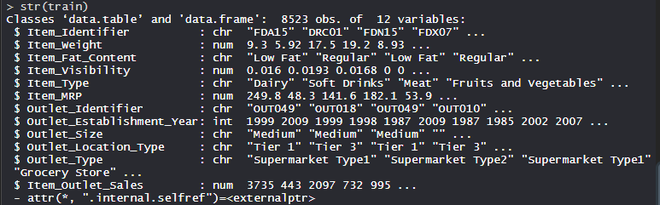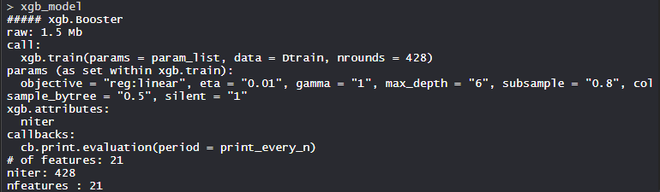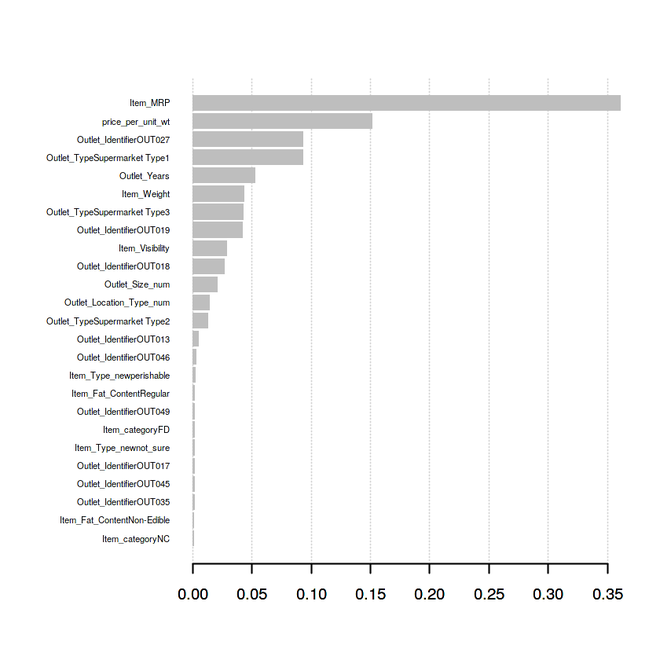Related Articles

# Feature Engineering in R Programming

• Last Updated : 28 Jun, 2021

Feature engineering is the most important technique used in creating machine learning models. Feature Engineering is a basic term used to cover many operations that are performed on the variables(features)to fit them into the algorithm. It helps in increasing the accuracy of the model thereby enhances the results of the predictions. Feature Engineered machine learning models perform better on data than basic machine learning models. The following aspects of feature engineering are as follows:

1.  Feature Scaling: It is done to get the features on the same scale( for eg. Euclidean distance).
2.  Feature Transformation: It is done to normalize the data(feature) by a function.
3.  Feature Construction: It is done to create new features based on original descriptors to improve the accuracy of the predictive model.
4.  Feature Reduction. : It is done to improve the statistical distribution and accuracy of the predictive model.

## Theory

The feature Construction method helps in creating new features in the data thereby increasing model accuracy and overall predictions. It is of two types:

1. Binning: Bins are created for continuous variables.
2. Encoding: Numerical variables or features are formed from categorical variables.

### Binning

Binning is done to create bins for continuous variables where they are converted to categorical variables. There are two types of binning: Unsupervised and Supervised.

• Unsupervised Binning involves Automatic and Manual binning. In Automatic Binning, bins are created without human interference and are created automatically.  In Manual Binning, bins are created with human interference and we specify where the bins to be created.
• Supervised Binning involves creating bins for the continuous variable while taking the target variable into the consideration also.

### Encoding

Encoding is the process in which numerical variables or features are created from categorical variables. It is a widely used method in the industry and in every model building process. It is of two types: Label Encoding and One-hot Encoding.

• Label Encoding involves assigning each label a unique integer or value based on alphabetical ordering. It is the most popular and widely used encoding.
• One-hot Encoding involves creating additional features or variables on the basis of unique values in categorical variables i.e. every unique value in the category will be added as a new feature.

## Implementation in R

### The Dataset

BigMart dataset consists of 1559 products across 10 stores in different cities. Certain attributes of each product and store have been defined. It consists of 12 features i.e Item_Identifier( is a unique product ID assigned to every distinct item), Item_Weight(includes the weight of the product), Item_Fat_Content(describes whether the product is low fat or not), Item_Visibility(mentions the percentage of the total display area of all products in a store allocated to the particular product), Item_Type(describes the food category to which the item belongs), Item_MRP(Maximum Retail Price (list price) of the product), Outlet_Identifier(unique store ID assigned. It consists of an alphanumeric string of length 6), Outlet_Establishment_Year(mentions the year in which store was established), Outlet_Size(tells the size of the store in terms of ground area covered), Outlet_Location_Type(tells about the size of the city in which the store is located), Outlet_Type(tells whether the outlet is just a grocery store or some sort of supermarket) and Item_Outlet_Sales( sales of the product in the particular store).

## R

 `# Loading data ``train = ``fread``(``"Train_UWu5bXk.csv"``) ``test = ``fread``(``"Test_u94Q5KV.csv"``) ` `# Structure ``str``(train)`

Output:### Performing Feature Engineering on the dataset

Using the Feature Construction method on the dataset which includes 12 features with 1559 products across 10 stores in different cities.

## R

 `# Loading packages``library``(data.table) ``# used for reading and manipulation of data``library``(dplyr)      ``# used for data manipulation and joining``library``(ggplot2)    ``# used for ploting``library``(caret)      ``# used for modeling``library``(e1071)      ``# used for removing skewness``library``(corrplot)   ``# used for making correlation plot``library``(xgboost)    ``# used for building XGBoost model``library``(cowplot)    ``# used for combining multiple plots` `# Importing datasets``train = ``fread``(``"Train_UWu5bXk.csv"``) ``test = ``fread``(``"Test_u94Q5KV.csv"``) ` `# Structure of dataset``str``(train)` `# Setting test dataset``# Combining datasets``# add Item_Outlet_Sales to test data``test[, Item_Outlet_Sales := ``NA``] ``combi = ``rbind``(train, test)``  ` `# Missing Value Treatment``missing_index = ``which``(``is.na``(combi\$Item_Weight))``for``(i ``in` `missing_index){``  ``item = combi\$Item_Identifier[i]``  ``combi\$Item_Weight[i] = ``mean``(combi\$Item_Weight``                         ``[combi\$Item_Identifier == item], ``                         ``na.rm = T)``}` `# Feature Engineering``# Feature Transformation``# Replacing 0 in Item_Visibility with mean``zero_index = ``which``(combi\$Item_Visibility == 0)``for``(i ``in` `zero_index){``  ``item = combi\$Item_Identifier[i]``  ``combi\$Item_Visibility[i] = ``mean``(``    ``combi\$Item_Visibility[combi\$Item_Identifier == item],``    ``na.rm = T``  ``)``}` `# Feature Construction``# Create a new feature 'Item_Type_new'``perishable = ``c``(``"Breads"``, ``"Breakfast"``, ``"Dairy"``,``               ``"Fruits and Vegetables"``, ``"Meat"``, ``"Seafood"``)``non_perishable = ``c``(``"Baking Goods"``, ``"Canned"``, ``"Frozen Foods"``,``                   ``"Hard Drinks"``, ``"Health and Hygiene"``,``                   ``"Household"``, ``"Soft Drinks"``)` `combi[,Item_Type_new := ``ifelse``(Item_Type %``in``% perishable, ``"perishable"``,``                               ``ifelse``(Item_Type %``in``% non_perishable,``                                      ``"non_perishable"``, ``"not_sure"``))]`  `combi[,Item_category := ``substr``(combi\$Item_Identifier, 1, 2)]` `combi\$Item_Fat_Content[combi\$Item_category == ``"NC"``] = ``"Non-Edible"` `# Years of operation of Outlets``combi[,Outlet_Years := 2013 - Outlet_Establishment_Year]``combi\$Outlet_Establishment_Year = ``as.factor``(combi\$Outlet_Establishment_Year)` `# Price per unit weight``combi[,price_per_unit_wt := Item_MRP/Item_Weight]` `# Label Encoding``combi[,Outlet_Size_num := ``ifelse``(Outlet_Size == ``"Small"``, 0,``                                 ``ifelse``(Outlet_Size == ``"Medium"``, 1, 2))]` `combi[,Outlet_Location_Type_num := ``ifelse``(Outlet_Location_Type == ``"Tier 3"``, 0,``                                   ``ifelse``(Outlet_Location_Type == ``"Tier 2"``, 1, 2))]` `combi[, ``c``(``"Outlet_Size"``, ``"Outlet_Location_Type"``) := ``NULL``]` `# One-hot Encoding``ohe = ``dummyVars``(``"~."``, data = combi[,-``c``(``"Item_Identifier"``,``                                       ``"Outlet_Establishment_Year"``,``                                       ``"Item_Type"``)], fullRank = T)``ohe_df = ``data.table``(``predict``(ohe, combi[,-``c``(``"Item_Identifier"``,``                                           ``"Outlet_Establishment_Year"``,``                                           ``"Item_Type"``)]))` `combi = ``cbind``(combi[,``"Item_Identifier"``], ohe_df)` `# Removing Skewness``skewness``(combi\$Item_Visibility)``skewness``(combi\$price_per_unit_wt)` `combi[,Item_Visibility := ``log``(Item_Visibility + 1)]``combi[,price_per_unit_wt := ``log``(price_per_unit_wt + 1)]` `# Scaling and Centering data``# index of numeric features``num_vars = ``which``(``sapply``(combi, is.numeric))``num_vars_names = ``names``(num_vars)` `combi_numeric = combi[,``setdiff``(num_vars_names,``                               ``"Item_Outlet_Sales"``), with = F]` `prep_num = ``preProcess``(combi_numeric, method=``c``(``"center"``, ``"scale"``))``combi_numeric_norm = ``predict``(prep_num, combi_numeric)` `# Transforming Features``combi[,``setdiff``(num_vars_names, ``"Item_Outlet_Sales"``) := ``NULL``]` `combi = ``cbind``(combi, combi_numeric_norm)` `# Splitting data``train = combi[1:``nrow``(train)]``test = combi[(``nrow``(train) + 1):``nrow``(combi)]` `# Removing Item_Outlet_Sales``test[,Item_Outlet_Sales := ``NULL``]` `# Model Building - xgboost``para_list = ``list``(``        ``objective = ``"reg:linear"``,``        ``eta=0.01,``        ``gamma = 1,``        ``max_depth=6,``        ``subsample=0.8,``        ``colsample_bytree=0.5``        ``)` `# D Matrix``d_train = ``xgb.DMatrix``(data = ``as.matrix``(train[,-``c``(``"Item_Identifier"``,``                                                 ``"Item_Outlet_Sales"``)]),``                      ``label= train\$Item_Outlet_Sales)``d_test = ``xgb.DMatrix``(data = ``as.matrix``(test[,-``c``(``"Item_Identifier"``)]))` `# K-fold cross validation``set.seed``(123) ``# Setting seed``xgb_cv = ``xgb.cv``(params = para_list,``               ``data = d_train,``               ``nrounds = 1000,``               ``nfold = 5,``               ``print_every_n = 10,``               ``early_stopping_rounds = 30,``               ``maximize = F)` `# Training model``model_xgb = ``xgb.train``(data = d_train,``                      ``params = para_list,``                      ``nrounds = 428)` `model_xgb` `# Variable Importance Plot``variable_imp = ``xgb.importance``(feature_names = ``setdiff``(``names``(train),``                              ``c``(``"Item_Identifier"``, ``"Item_Outlet_Sales"``)),``                              ``model = model_xgb)` `xgb.plot.importance``(variable_imp)`

Output:

• Model model_xgb:The XgBoost model consists of 21 features with the objective of regression linear, eta is 0.01, gamma is 1, max_depth is 6, colsample_bytree = 0.5, and silent is 1.

• Variable Importance plot:price_per_unit_wt is the second most important variable or feature for the predictive model followed by Outlet_Years being the sixth most important variable or feature for the predictive model. Item_category, Item_Type_new features played a major role in improving the predictive model and thus improving model accuracy. So, Feature Engineering is the most important method for building an efficient, scalable, and accurate predictive model.

My Personal Notes arrow_drop_up